# List.Accumulate Hidden Gem of Power Query List Functions in Power BIThere are a number of List transformations available in Power Query’s graphical interface. However, the number of functions in the graphical interface is very limited. In this post, I’m going to explain about a function that is really powerful and is not yet listed in the graphical interface. List.Accumulate is a function that loops through items of a list and applies a transformation. This function at the time of writing this post is only available through Power Query M scripting. If you like to learn more about Power BI read Power BI book from Rookie to Rock Star.

## List Transformations in Graphical Interface of Power Query

If you have a list, you can see what transformations are available to be applied to it using the graphical interface.  you can create a list with a simple M script as below;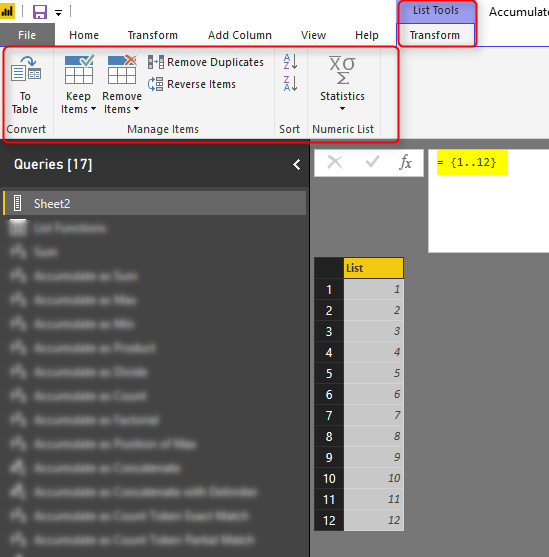This code generates a list of numbers from 1 to 12. Now you can see in the top menu under List Tools, that there are a number of transformations available for List. These items are very few options such as; convert to a table, keep items, remove items, and etc. Altogether considering all options in every element this list is not more than 20 functions. However, let’s look at the number of List functions in M script.

## List Functions in M; Power Query Formula Language

I have written previously about the usage of the #shared key to finding all functions available in M. you can then filter it to only “List.” Functions and then you will end up with a bit list of functions; 69 functions! more than 3 times of what you see in the graphical interface.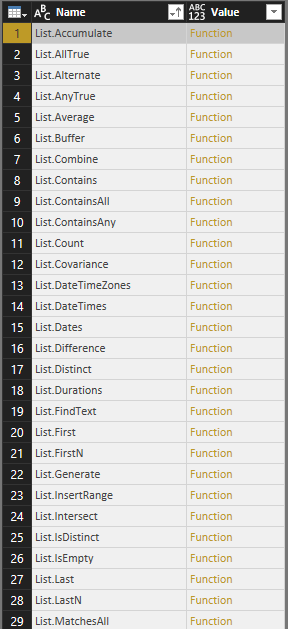Some of these functions Some of the functions in this list are very useful and powerful. Example of those functions is List.Dates, List.Numbers, List.Generate, List.Accumulate and many others. Obviously, we cannot go through all functions in one blog post. In this post, I’ll be covering List.Accumulate and in future posts, I’ll talk about other functions.

## List.Accumulate Function

List.Accumulate is a function that can easily save a number of steps in your Power Query transformations, instead of applying multiple steps, you can simply use List.Accumulate to overcome what you want. List.Accumulate function loops through the list and accumulate a value as a result. This function needs usually three parameters; the list itself, seed, and accumulator. Here are parameters explained in details;

best way to learn about seed and accumulator is through some examples, let’s apply some transformations with List.Accumulate and see how these two parameters are working.

## Accumulate to Calculate Sum

The sum is a function that basically is accumulating every two values in the list till the end of the list. if you want to write Sum with List.Accumulate, you can do it with this expression:

The function part of this expression is: (state, current)=>state+current

state is the value accumulated in the calculation. current is the current item in the list. seed is the initial value of the state

Let’s see how the calculation works. To clarify it more in details, I explained the value of state, current, and accumulator in every step;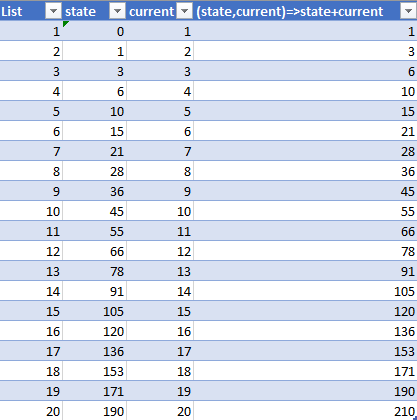List.Accumulate loops through every item in the list and run accumulator function. the very first time, the state value is equal to seed. which in this case is zero. current is the current value in the list. For the very first item that value is 1. so accumulator result is state+current=0+1=1. this value then will be the state of the next item on the list. for the next item, the state is 1 (calculated from the previous row), and current is 2. state+current becomes 1+2=3. this process continues through the whole list, so the final state value for a list from 1 to 20, becomes 210, which is equal to the sum of those values. In every row of the list, we added that to the previous row’s result.

## Accumulate to Calculate Max

learning how the accumulate function can cover basic tasks, help you to understand how accumulator function works. for applying Max, you need to compare every two items in the list and pick the one which is bigger. Here is the script;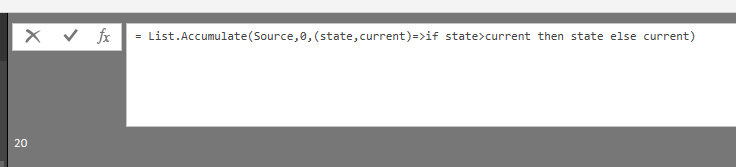remember the same thing about the state, current, and seed, this is how the calculation works;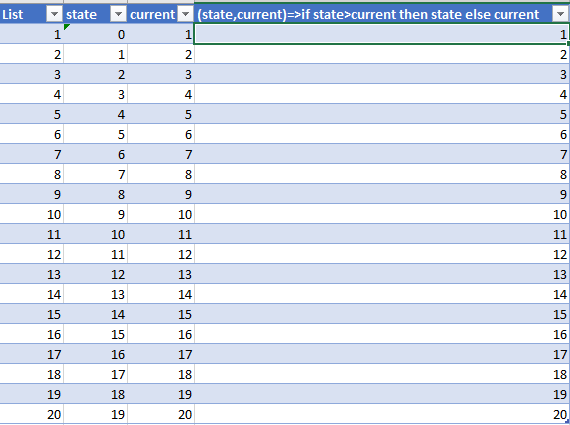the function (state, current)=>if state>current then state else current will run on every row and give you the result. Remember that seed value should be a value less than any other values for this case.

## Accumulate as Product or Divide

you can use the same logic with a different accumulator to calculate Product or Divide, Here is the calculation for Product;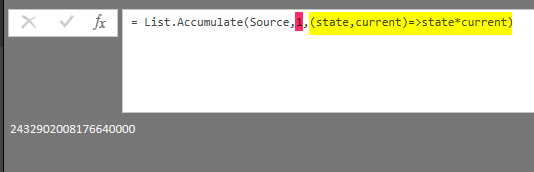for Product or Divide, you need to set the seed as 1, because if it is zero, then divide or multiply considering zero will end up zero always. Here is the calculation for Divide;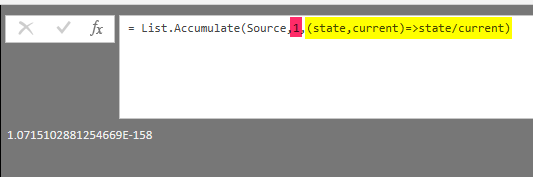## Accumulate as Count

Count of a list is basically the number of items in a list. this can be achieved with no need of the current item, just using seed and state as below;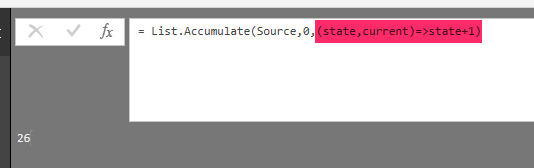in every row, the value would be plus one of the state value from previous row’s calculation. as a result, you get the count.

## Accumulate as Concatenate (with delimiter or without)

So far, we applied accumulate on lists which had a number in every item. Now, let’s apply it to a list of text items. To concatenate two items you just need to add them one after each other with concatenation character which is the ampersand (&).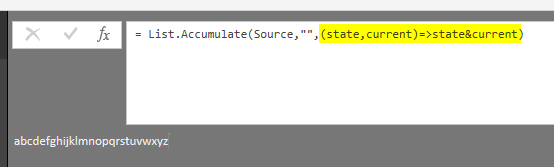This worked on a list of items which are text values {“a”..”z”}, and the result is the concatenation of all items in the list. Please note that the seed, in this case, is an empty text.

if you want to add a delimiter between items, you can add the delimiter plus a condition to check if this is the very first item or not.## Accumulate as Count Token Exact Match

now that you’ve learned the logic of the accumulator, you can apply it to do any expressions. For example, if you want to calculate count of items in the list which their value matches exactly with a value, you can write this expression;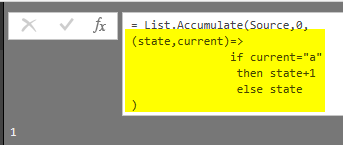the logic is simple, if the item matches with “a” (which in this case is our token), then count it, otherwise don’t

## Accumulate as Count Token Partial Match

Similar to the previous calculation, however this time we want to count the item, even it partially matches the text. this can be done with the help of Text.Contains function. and because of Text.Contains might not find the lower case or upper case matches, we convert it to lower case beforehand.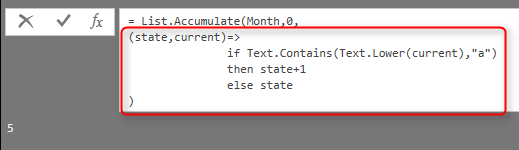## Accumulate as Conditions on Records

So far, we went through a lot of use cases and examples of List.Accumulate function. You understand that this function can be so powerful and useful in many scenarios. However, the main use cases of List.Accumulate is to apply to scenarios which other functions cannot resolve easily. List.Sum might be better used than List.Accumulate which only calculates the sum. However, there are many scenarios that a number of steps are needed to get the result you want with normal functions. In those cases, List.Accumulate is your friend.

For example; consider the situation that you have a list, and this list is a list of records! every record might have a different set of fields, you want to fetch only records that have a specific field on their list, and get their position as a concatenated result. This process, using other list functions might take a number of steps, however, with List.Accumulate that is easy.

Here is the sample input list;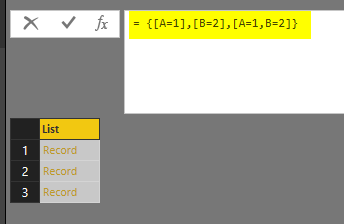As you can see the list includes records, and to find out what each record has, you need to expand it. a list of records cannot be expanded because it will add the number of columns and list can have only one column. so you have to convert it to a table, and then expand it. As you feel now; there are a number of steps required to get the result we wanted. However, List.Accumulate can be a big help in this scenario. Here is the calculation with List.Accumulate;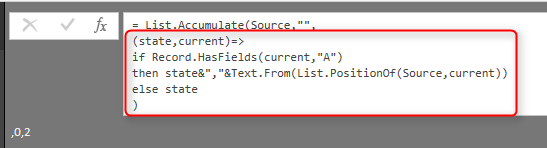Record.HasFields used to determine if a record contains a field (“A” in this example).

List.PositionOf used to get the position of that record which satisfy the criteria above.

## Summary

This post explained how List.Accumulate works. List.Accumulate is a very powerful function, that can easily save a number of steps in your Power Query transformations. In this post, you’ve learned basics of this function with using it for simple operations such as Sum, Divide, Product, Max, Count and etc. You also learned that the main power of this function is when basic functions cannot operate easily. You learned that the accumulator function gives you full power to write exactly what you want. In future posts, I’ll write about other List functions that can be very powerful, but you still don’t have it in the graphical interface.

Have you liked the List.Accumulate function as I do? if yes, please share your story that how this function can be helpful for you in the comments below.

Trainer, Consultant, Mentor
Reza Rad is a Microsoft Regional Director, an Author, Trainer, Speaker and Consultant. He has a BSc in Computer engineering; he has more than 20 years’ experience in data analysis, BI, databases, programming, and development mostly on Microsoft technologies. He is a Microsoft Data Platform MVP for nine continuous years (from 2011 till now) for his dedication in Microsoft BI. Reza is an active blogger and co-founder of RADACAD. Reza is also co-founder and co-organizer of Difinity conference in New Zealand.
His articles on different aspects of technologies, especially on MS BI, can be found on his blog: https://radacad.com/blog.
He wrote some books on MS SQL BI and also is writing some others, He was also an active member on online technical forums such as MSDN and Experts-Exchange, and was a moderator of MSDN SQL Server forums, and is an MCP, MCSE, and MCITP of BI. He is the leader of the New Zealand Business Intelligence users group. He is also the author of very popular book Power BI from Rookie to Rock Star, which is free with more than 1700 pages of content and the Power BI Pro Architecture published by Apress.
He is an International Speaker in Microsoft Ignite, Microsoft Business Applications Summit, Data Insight Summit, PASS Summit, SQL Saturday and SQL user groups. And He is a Microsoft Certified Trainer.
Reza’s passion is to help you find the best data solution, he is Data enthusiast.

## 3 thoughts on “List.Accumulate Hidden Gem of Power Query List Functions in Power BI”

•MarcelBeug says:

Small correction: seed is a required parameter; It may be null, but it is not optional.
Coincidentally I just provided a List.Accumulate solution for an Inventory flow question on Stackoverflow: https://stackoverflow.com/questions/47824613/network-flow-database-in-power-query-table
In this case, List.Accumulate builds a list of records with 3 fields each.
=
List.Accumulate(
List.Skip(IncomingSupplies),
{[Beginning On Hand Inventory = Source[Starting Inventory]{0},
Shipments = 0.5 * #”Beginning On Hand Inventory”,
Ending On Hand = #”Beginning On Hand Inventory” + IncomingSupplies{0} – Shipments]},
(Result,Supply) =>
Result &
{[Beginning On Hand Inventory = List.Last(Result)[Ending On Hand],
Shipments = 0.5 * #”Beginning On Hand Inventory”,
Ending On Hand = #”Beginning On Hand Inventory” + Supply – Shipments]}),

•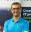Reza Rad says:

Hi Marcel,
Very good catch. it was a type. thanks for finding it 🙂 really appreciate your comment.
Also very good example for List.Accumulate you used 🙂
Cheers
Reza